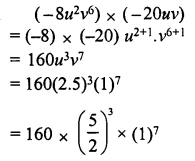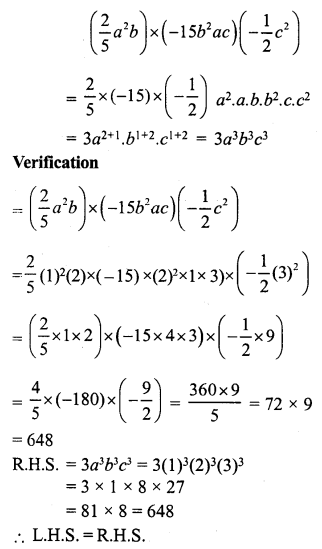# RS Aggarwal Class 7 Solutions Chapter 6 Algebraic Expressions Ex 6B

In this chapter, we provide RS Aggarwal Solutions for Class 7 Chapter 6 Algebraic Expressions Ex 6B for English medium students, Which will very helpful for every student in their exams. Students can download the latest RS Aggarwal Solutions for Class 7 Chapter 6 Algebraic Expressions Ex 6B Maths pdf, free RS Aggarwal Solutions Class 7 Chapter 6 Algebraic Expressions Ex 6B Maths book pdf download. Now you will get step by step solution to each question.

### RS Aggarwal Solutions for Class 7 Chapter 6 Exponents Ex 6B Download PDF

Question 1.
Solution:
3 x 8 a2+4 = 24a6

Question 2.
Solution:
(-6x3) x (5x2) = -6 x 5x2+3 = -30x5

Question 3.
Solution:
(-4ab) x (-3a2bc)
= (-4) x (-3) a. a2.b. b. c
= 12.a2+1. b1+1.c
= 12a3b2c

Question 4.
Solution:
= (2a2b3) x (-3a3b)
= 2 x (-3) a2. a3. b3. b. b
= -6a2+3.b3+1
= -6a5.b4

Question 5.
Solution:Question 6.
Solution:Question 7.
Solution:Question 8.
Solution:Question 9.
Solution:Question 10.
Solution:Question 11.
Solution:Question 12.
Solution:Question 13.
Solution:Question 14.
Solution:Question 15.
Solution:Question 16.
Solution:Question 17.
Solution:Question 18.
Solution:Question 19.
Solution:Question 20.
Solution:Question 21.
Solution:Find the products given below and in each case verify the result for a = 1, b = 2 and c = 3 :
Question 22.
Solution:Question 23.
Solution:Question 24.
Solution:Question 25.
Solution:All Chapter RS Aggarwal Solutions For Class 7 Maths

—————————————————————————–

All Subject NCERT Exemplar Problems Solutions For Class 7

All Subject NCERT Solutions For Class 7

*************************************************

I think you got complete solutions for this chapter. If You have any queries regarding this chapter, please comment on the below section our subject teacher will answer you. We tried our best to give complete solutions so you got good marks in your exam.

If these solutions have helped you, you can also share rsaggarwalsolutions.in to your friends.